Derivatives on the AP Calculus AB & BC Exams: A Refresher

When studying for the AP Calculus AB or BC exams, being comfortable with derivatives is extremely important. They will come up in almost every problem, both on the AB and BC exams. And these problems will rely not only on understanding how to take the derivatives of a variety of functions, but also on understanding how a derivative works, and where it comes from.

Definition of a Derivative

This is the perfect place to start. What is a derivative? A derivative of a function is a representation of the rate of change of one variable in relation to another at a given point on a function. In two dimensions, it gives an equation for the slope of a tangent line of a function at a point.

It is very important to remember that not all functions are continuously differentiable. To be differentiable in a certain domain, a function must be continuous and have no sharp points. If there is a break in the graph (such as in a piecewise function, or where we might have vertical asymptotes), or if there is a sharp bend (such as absolute value problems), we cannot find a derivate in that interval.

i.e. y= |x| is not differentiable at x = 0 because there is a sharp bend.y=cos⁡(x) is differentiable at x = 0 because there is no sharp bend, and it is continuous (there is only one possible slope of the tangent line at a point).How to Calculate the Derivative

If we remember from algebra, the definition of a slope is m= (y2-y1)/(x2-x1). This takes two points (x1, y1 and x2, y2) but on a curve, we only have one. Therefore, to find our derivative, we are imagining what is happening as our second point becomes closer and closer to our first point – to the point where the distance between them is infinitely small. This is our derivative.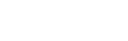The above formula gives us our derivative of a function f at a. It is important to understand the above formula and how it is used to determine a derivative.

AP Calculus Exam Review: Derivative Notation

There are several different manners for writing derivatives that you might come across. The first two below are the most common, and you will see both on the AP Calculus exams. Newton’s notation is more often used in physics classrooms, so while it is mentioned below, it is not often seen on the AP exams. There are several other notations as well, but they are rarely seen and you won’t find them on the AP exams.

Leibniz’s Notation:Lagrange’s Notation:Newton’s Notation: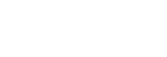Common Derivatives

I’ve written two lists of common derivatives (these are certainly not exhaustive lists). The first includes the most common functions, which will come up repeatedly. You should have these thoroughly memorized; to succeed on the AP exams, these are the functions you should not need to think twice about. The second list contains derivatives which are slightly less common, but should still be at your disposal.

More common:Less common:Derivative Rules

The following is a list of common derivative rules which you will use. It is imperative that you are facile with all of these before the AP exam. You should know exactly which one to use when, and how each can be implemented.

Product Rule: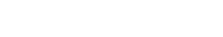Quotient Rule: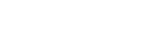Power Rule: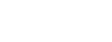Chain Rule:We will have much more on our blog about derivatives plus lots of practice problems, soon.
This PDF printout of common derivatives and integrals you can put in your notes and refer to as you study.

Improve your SAT or ACT score, guaranteed. Start your 1 Week Free Trial of Magoosh SAT Prep or your 1 Week Free Trial of Magoosh ACT Prep today!Author

•Zachary is a former mechanical engineer and current high school physics, math, and computer science teacher. He graduated from McGill University in 2011 and spent time in the automotive industry in Detroit before moving into education. He has been teaching and tutoring for the past five years, but you can also find him adventuring, reading, rock climbing, and traveling whenever the opportunity arises.